Download PDF文章快速检索 高级检索

, 周钢2 , 刘晓梅3 , 翁史烈11. 上海交通大学 机械与动力工程学院, 上海 200240;
2. 上海交通大学 数学系, 上海 200240;
3. 上海第二工业大学 理学院, 上海 201209

Symplectic weighted discontinuous Galerkin method with minimal phase-lag
, ZHOU Gang2 , LIU Xiaomei3 , WENG Shilie11. School of Mechanical Engineering, Shanghai Jiao Tong University, Shanghai 200240, China ;
2. Department of Mathematics, Shanghai Jiao Tong University, Shanghai 200240, China ;
3. Department of Mathematics, Shanghai Second Polytechnic University, Shanghai 201209, China
Received: 2015-08-10; Accepted: 2015-11-13; Published online: 2015-12-17 14:41
Foundation item: National Natural Science Foundation of China (50876066); Shanghai University Youth Teacher Training Program (ZZZZEGD15007); Shanghai Second Polytechnic University Fund Project (EGD15XQD14); Shanghai Second Polytechnic University Applied Mathematics Key Discipline (XXKZD1304)
Corresponding author. WENG Shilie, E-mail:slweng@sjtu.edu.cn
Abstract: Symplectic finite difference method (FDM) can keep the symplectic structure, and finite element method (FEM) can keep the symplectic structure as well as energy conservation for linear Hamiltonian systems. However, symplectic FDM and FEM still have phase errors for the numerical solution, so, the computational accuracy is not very well in time domain analysis. Symplectic weighted discontinuous Galerkin method with minimal phase-lag (WDG-PF) is proposed for Hamiltonian systems. This method is symplectic and can highly decrease the phase error, compared to traditional method for Hamiltonian systems. Meanwhile, WDG-PF can keep the conservation of energy as well as the symplectic structure of Hamiltonian systems. WDG-PF can solve the phase-lag problem of continuous Galerkin method, and WDG is symplectic by the technique of weight. Compared to symmetric symplectic(FSJS) algorithm, Runge-Kutta-Nystrom(SRKN) and symplectic partitioned Runge-Kutta (SPRK) methods which are aimed at increasing the accuracy of phase error, WDG-PF ismuch more accurate and increase the energy accuracy of Hamiltonian systems, tremedously. The phase error and Hamiltonian function error almost achieve the accuracy of computer. WDG-PF has the ultraconvergence point in each element. Especially, for the systems with high and low frequency signals, and seldom has a method can simulate the high and low frequency signals with a fixed time step, WDG-PF can effectively simulate the high and low frequency signals with large time step. The numerical experiments show its validity.
Key words: Hamiltonian systems     discontinuous Galerkin method     phase error     symplectic algorithm     energy-preserving

1 Hamilton系统及相位误差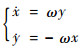(1)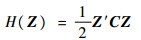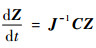(2)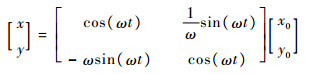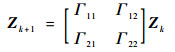(3)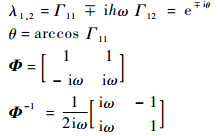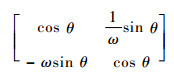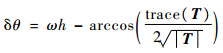(4)

2 加权间断有限元方法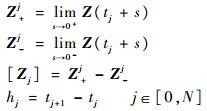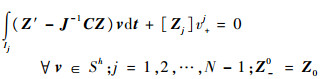(5)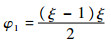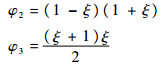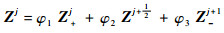(6)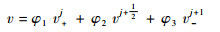(7)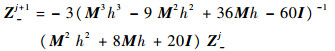(8)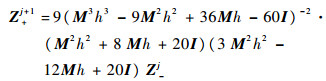(9)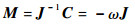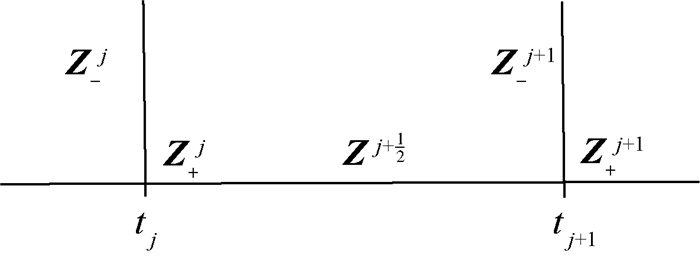图 1 计算过程 Fig. 1 Computational procedure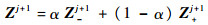(10)

3 极小化相位误差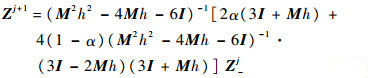(11)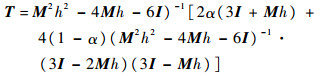(12)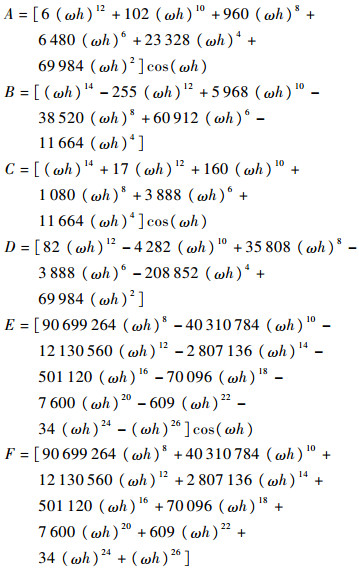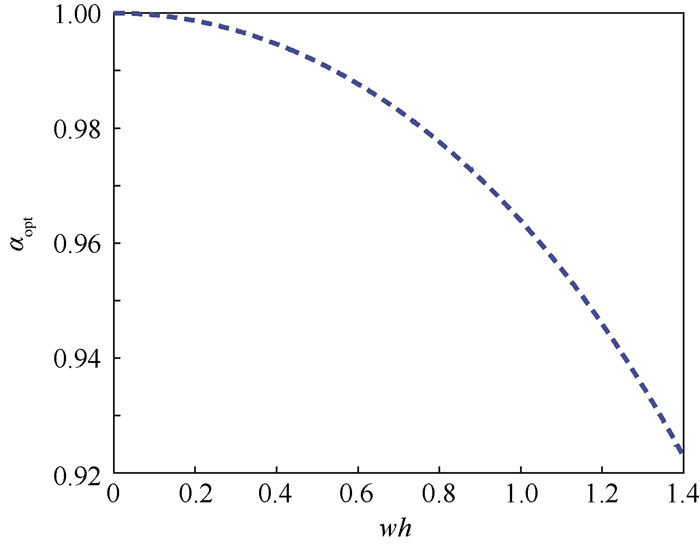图 2 ωh和最优权重αopt的关系 Fig. 2 Relationship between ωh and αopt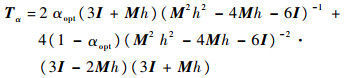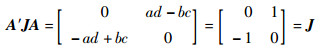A是辛矩阵。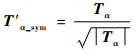(13)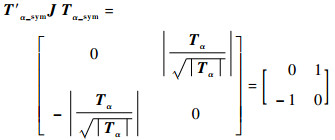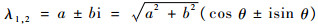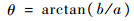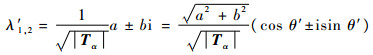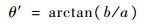θ=θ′，所以TαTα_sym具有相同的相位误差。即对极小化相位误差的矩阵Tα进行式(13)的变换，得到新的Jacobi矩阵Tα_sym同样具有极小的相位误差。

4 数值算例 4.1 椭圆型Hamilton系统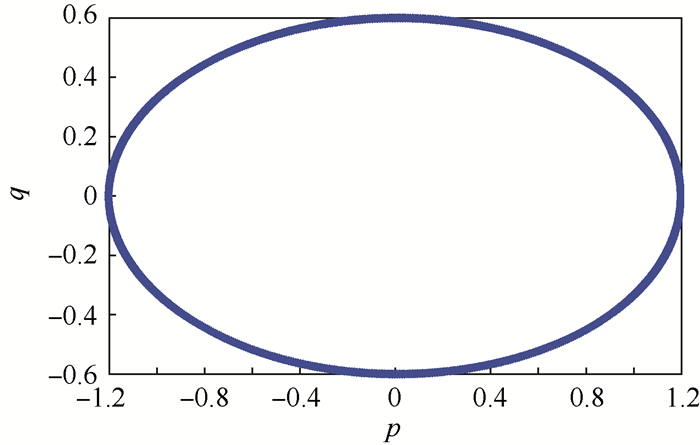图 3 相图 Fig. 3 Phase portrait

 参数 误差绝对值的最大值 WDG-PF TFE2  AVF  p(t) 9.7382×10 -15 2.3999 2.3660 q(t) 9.6202×10 -17 0.1461 1.7997 能量 3.3061×10 -38 1.3767×10 -14 0

 参数 极小相位误差 WDG-PF FSJS SPRK RKN p(t) 9.7382×10-15 0.1859 0.0072 0.8988 q(t) 9.6202×10-17 0.1084 0.0121 0.0030 能量 3.3061×10-38 0.0549 0.0263 1.3493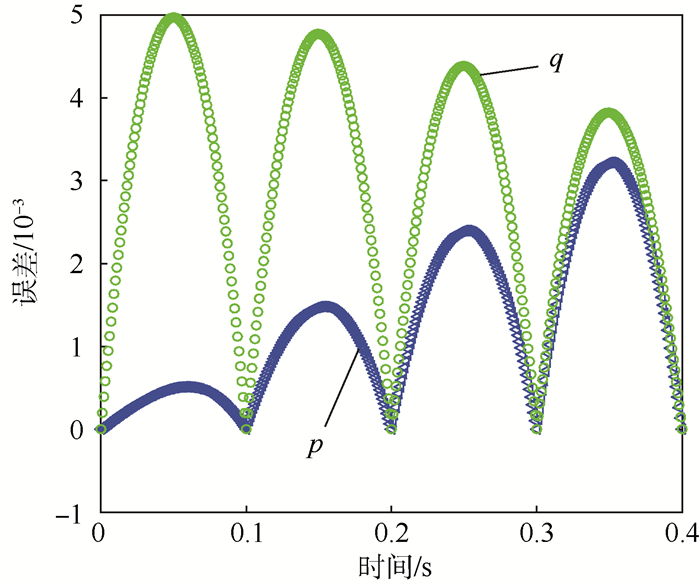图 4 一次加权间断元方法在前4个单元内部误差图(h=0.1) Fig. 4 Error distribution of one-order WDG-PF in first four elements(h=0.1)

4.2 高低混频Hamilton系统

Hamilton函数：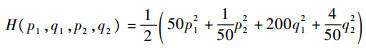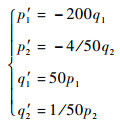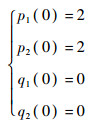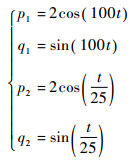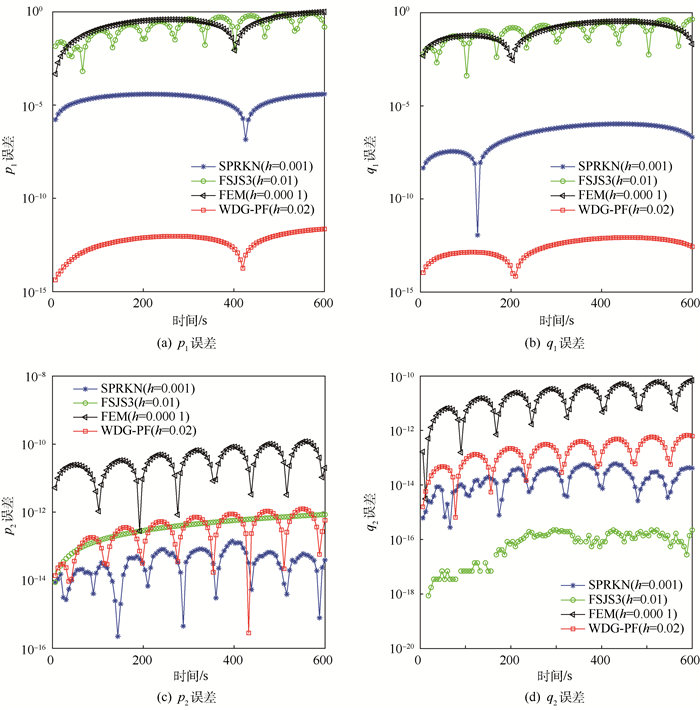图 5 几种不同方法求解p1、q1、p2和q2误差 Fig. 5 Errors of p1, q1, p2 and q2 solved by different methods

 方法 能量(误差) t=200 s t=400 s t=600 s WDG-PF 1.919×10-12 4.019×10-12 5.983×10-12 TFE1 9.164×10-11 1.213×10-10 2.7×10-10 FSJS 0.143 0.019 44 0.085 56 SPRK 6.998×10-5 4.388×10-5 4.294×10-5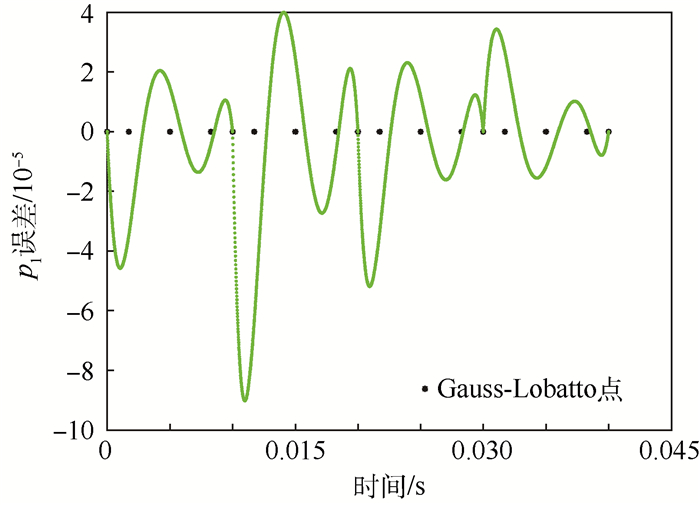图 6 4次WDG-PF在前4个单元内部误差情况 Fig. 6 Error distribution for four-order WDG-PF in first four elements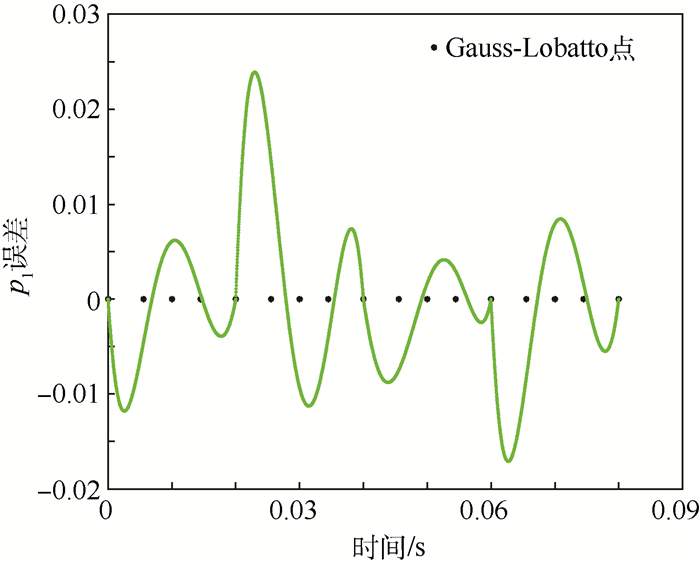图 7 3次WDG-PF在前4个单元内部误差情况 Fig. 7 Error distribution for third-order WDG-PF in first four elements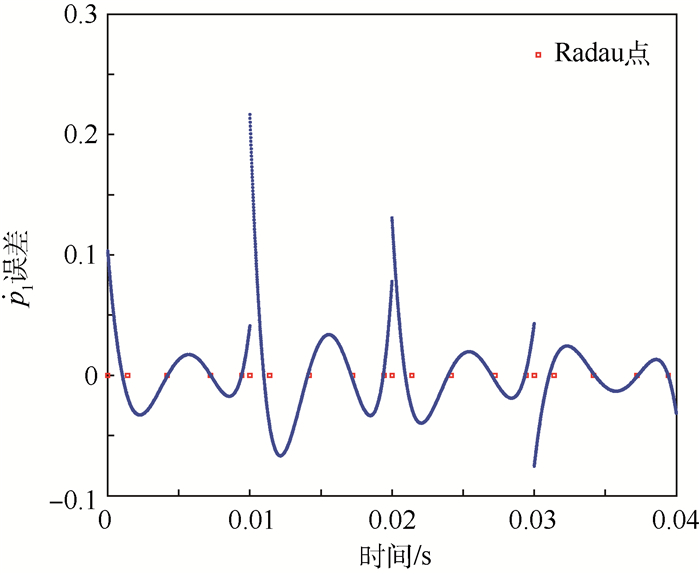图 8 4次WDG-PF在前4个单元内部导数误差情况 Fig. 8 Derivative error distribution for four-order WDG-PF in first four elements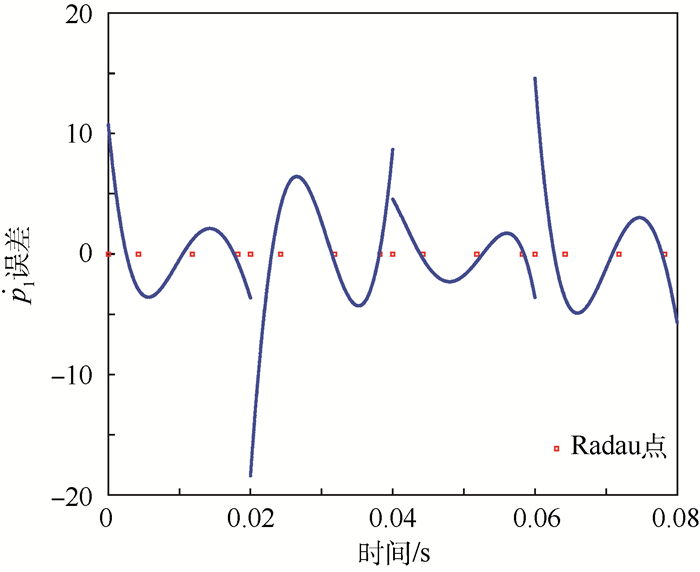图 9 3次WDG-PF在前4个单元内部导数误差情况 Fig. 9 Derivative error distribution for third-order WDG-PF in first four elements

5 结论

1) 对于给定的Hamilton系统和单元长度，使得相位误差极小且保辛，权重α“一次计算，终身使用”。

2) 可以解决间断有限元方法不能保证Hamilton系统辛结构的缺点，具有极小的相位误差和极高精度的保能量特性，几乎达到计算机精度。

3) 这种加权间断有限单元有超收敛点。

4) 特别针对高低混频Hamilton系统或者刚性问题，WDG-PF方法可以在大步长下同时实现对高频和低频信号的准确仿真。

  FENG K. Difference schemes for Hamiltonian formalism andsymplectic geometry[J]. Journal of Computational Mathematics, 1986, 4 (3) : 279 –289.  FENG K., QIN M. Symplectic geometric algorithms for Hamiltonian systems[M]. Heidelberg: Springer, 2010 : 279 -289.  CALVO M P. Numerical Hamiltonian problems[M]. London: CRC Press, 1994 : 315 -356.  BRUSA L, NIGRO L. A one-step method for direct integration of structural dynamic equations[J]. International Journal for Numerical Methods in Engineering, 1980, 15 (5) : 685 –699. DOI:10.1002/(ISSN)1097-0207  邢誉峰, 杨蓉. 单步辛算法的相位误差分析及修正[J]. 力学学报, 2007, 39 (5) : 668 –671. XING Y F, YANG R. Phase errors and their correction in symplectic implicit single-step algorithm[J]. Chinese Journal of Theoretical and Applied Mechanics, 2007, 39 (5) : 668 –671. (in Chinese)  邢誉峰, 冯伟. 李级数算法和显式辛算法的相位分析[J]. 计算力学学报, 2009, 26 (2) : 167 –171. XING Y F, FENG W. Phase analysis of Lie series algorithm and explicit symplectic algorithm[J]. Chinese Journal of Computational Mechanics, 2009, 26 (2) : 167 –171. (in Chinese)  陈璐, 王雨顺. 保结构算法的相位误差分析及其修正[J]. 计算数学, 2014, 36 (3) : 271 –290. CHEN L, WANG Y S. Phase error analysis and correction of structure preserving algorithms[J]. Mathematica Numerica Sinica, 2014, 36 (3) : 271 –290. (in Chinese)  VYVER H V D. A symplectic Runge-Kutta-Nystrom method with minimal phase-lag[J]. Physics Letters A, 2007, 367 (1) : 16 –24.  MONOVASILIS T, KALOGIRATOU Z, SIMOS T E. Exponentially fitted symplectic runge-Kutta-Nyströmethods[J]. Applied Mathematics & Information Sciences, 2013, 7 (1) : 81 –85.  MONOVASILIS T, KALOGIRATOU Z, SIMOS T E. Symplectic Partitioned Runge-Kutta methods with minimal phase-lag[J]. Computer Physics Communications, 2010, 181 (7) : 1251 –1254. DOI:10.1016/j.cpc.2010.03.013  SIMOS T E. A two-step method with vanished phase-lag and its first two derivatives for the numerical solution of the Schr dinger equation[J]. Journal of Mathematical Chemistry, 2011, 49 (10) : 2486 –2518. DOI:10.1007/s10910-011-9897-1  MONOVASILIS T, KALOGIRATOU Z, SIMOS T E. Two new phase-fitted symplectic partitioned Runge-Kutta methods[J]. International Journal of Modern Physics C, 2011, 22 (12) : 1343 –1355. DOI:10.1142/S0129183111016932  刘晓梅, 周钢, 王永泓, 等. 辛算法的纠飘研究[J]. 北京航空航天大学学报, 2013, 39 (1) : 22 –26. LIU X M, ZHOU G, WANG Y H, et al. Rectifying drifts of symplectic algorithm[J]. Journal of Beijing University of Aeronautics and Astronautics, 2013, 39 (1) : 22 –26. (in Chinese)  TANG W, SUN Y. Time finite element methods:A unified framework for numerical discretizations of ODEs[J]. Applied Mathematics & Computation, 2012, 219 (4) : 2158 –2179.  汤琼, 陈传淼. Hamilton系统的连续有限元法[J]. 应用数学和力学, 2007, 28 (8) : 958 –966. TANG Q, CHEN C M. Continuous finite element methods of Hamiltonian systems[J]. Applied Mathematics and Mechanics, 2007, 28 (8) : 958 –966. (in Chinese)  陈传淼, 汤琼. Hamilton系统的有限元研究[J]. 数学物理学报, 2011, 31A (1) : 18 –33. CHEN C M, TANG Q. Study of finite element for Hamiltonian systems[J]. Acta Mathematica Scientia, 2011, 31A (1) : 18 –33. (in Chinese)  HU S F, CHEN C M. Runge-Kutta method finite element method and regular algorithms for Hamiltonian system[J]. Applied Mathematics & Mechanics:English Edition, 2013, 34 (6) : 747 –760.  LI C H, CHEN C M. Ultraconvergence for averaging discontinuous finite elements and its applications in Hamiltonian system[J]. Applied Mathematics & Mechanics:English Edition, 2011, 32 (7) : 943 –956.  DELFOUR M, HAGER W, TROCHU F. Discontinuous Galerkin methods for ordinary differential equations[J]. Mathematics of Computation, 1981, 36 (154) : 455 –473. DOI:10.1090/S0025-5718-1981-0606506-0  WANG D, XIAO A, LI X. Parametric symplectic partitioned Runge-Kutta methods with energy-preserving properties for Hamiltonian systems[J]. Computer Physics Communications, 2013, 184 (2) : 303 –310. DOI:10.1016/j.cpc.2012.09.012  QUISPEL G R W, MCLAREN D I. A new class of energy-preserving numerical integration methods[J]. Journal of Physics A Mathematical & Theoretical, 2008, 41 (4) : 75 –97.

#### 文章信息

ZHU Shuai, ZHOU Gang, LIU Xiaomei, WENG Shilie

Symplectic weighted discontinuous Galerkin method with minimal phase-lag

Journal of Beijing University of Aeronautics and Astronsutics, 2016, 42(8): 1682-1690
http://dx.doi.org/10.13700/j.bh.1001-5965.2015.0523